# Complex Numbers

Michael Fowler

### Real Numbers

Let us think of the ordinary numbers as set out on a line which goes to infinity in both positive and negative directions.  We could start by taking a stretch of the line near the origin (that is, the point representing the number zero) and putting in the integers as follows: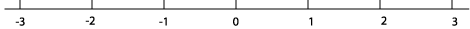Next, we could add in rational numbers, such as $½$ ,  23/11, etc., then the irrationals like $\sqrt{2},$

then numbers like $\pi ,$ and so on, so any number you can think of has its place on this line.

Now let’s take a slightly different point of view, and think of the numbers as represented by a vector from the origin to that number, so 1 is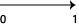and, for example,  $–$2 is represented by: Fig 3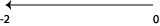Note that if a number is multiplied by $–$1, the corresponding vector is turned through 180 degrees.  In pictures,The “vector” 2 is turned through $\pi ,$ or 180 degrees, when you multiply it by $–$1.

What are the square roots of 4?

Well, 2, obviously, but also $–$2, because multiplying the backwards pointing vector $–$2 by $–$2 not only doubles its length, but also turns it through 180 degrees, so it is now pointing in the positive direction.  We seem to have invented a hard way of stating that multiplying two negatives gives a positive, but thinking in terms of turning vectors through 180 degrees will pay off soon.

### Solving Quadratic Equations

In solving the standard quadratic equation

$a{x}^{2}+bx+c=0$

we find the solution to be:

$x=\frac{-b±\sqrt{{b}^{2}-4ac}}{2a}$.

The problem with this is that sometimes the expression inside the square root is negative.  What does that signify?  For some problems in physics, it means there is no solution.  For example, if I throw a ball directly upwards at 10 meters per sec, and ask when will it reach a height of 20 meters, taking $g=10$ m per sec2, the solution of the quadratic equation for the time $t$ has a negative number inside the square root, and that means that the ball doesn’t get to 20 meters, so the question didn’t really make sense.

We shall find, however, that there are other problems, in wide areas of physics, where negative numbers inside square roots have an important physical significance.  For that reason, we need to come up with a scheme for interpreting them.

The simplest quadratic equation that gives trouble is

${x}^{2}+1=0,$

the solutions being $x=±\sqrt{-1}.$

What does that mean?  We’ve just seen that the square of a positive number is positive, and the square of a negative number is also positive, since multiplying one negative number, which points backwards, by another, which turns any vector through 180 degrees, gives a positive vector.  Another way of saying the same thing is to regard the minus sign itself, -, as an operator which turns the number it is applied to through 180 degrees.  Now $\left(-2\right)×\left(-2\right)$ has two such rotations in it, giving the full 360 degrees back to the positive axis.

To make sense of the square root of a negative number, we need to find something which when multiplied by itself gives a negative number

Let’s concentrate for the moment on the square root of $–$1, from the quadratic equation above.  Think of $–$1 as the operator $–$ acting on the vector 1, so the $–$ turns the vector through 180 degrees.  We need to find the square root of this operator, the operator which applied twice gives the rotation through 180 degrees.  Put like that, it is pretty obvious that the operator we want rotates the vector 1 through 90 degrees.

But if we take a positive number, such as 1, and rotate its vector through 90 degrees only, it isn’t a number at all, at least in our original sense, since we put all known numbers on one line, and we’ve now rotated 1 away from that line. The new number created in this way is called a pure imaginary number, and is denoted by $i.$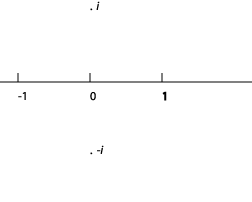Once we’ve found the square root of $–$1, we can use it to write the square root of any other negative number$—$for example, $2i$ is the square root of $–$4.  Putting together a real number from the original line with an imaginary number (a multiple of $i$ ) gives a complex number.   Evidently, complex numbers fill the entire two-dimensional plane.  Taking ordinary Cartesian coordinates, any point $P$ in the plane can be written as $\left(x,y\right)$ where the point is reached from the origin by going $x$ units in the direction of the positive real axis, then $y$ units in the direction defined by $i,$ in other words, the $y$ -axis.

Thus the point $P$ with coordinates $\left(x,y\right)$ can be identified with the complex number $z,$ where

$z=x+iy.$

The plane is often called the complex plane, and representing complex numbers in this way is sometimes referred to as an Argand Diagram.

Visualizing the complex numbers as two-dimensional vectors, it is clear how to add two of them together.  If ${z}_{1}={x}_{1}+i{y}_{1},$ and ${z}_{2}={x}_{2}+i{y}_{2},$ then

The real parts and imaginary parts are added separately, just like vector components.

Multiplying two complex numbers together does not have quite such a simple interpretation. It is, however, quite straightforward$—$ordinary algebraic rules apply, with ${i}^{2}$ replaced where it appears by $-1.$  So for example, to multiply ${z}_{1}={x}_{1}+i{y}_{1}$ by ${z}_{2}={x}_{2}+i{y}_{2},$

${z}_{1}{z}_{2}=\left({x}_{1}+i{y}_{1}\right)\left({x}_{2}+i{y}_{2}\right)=\left({x}_{1}{x}_{2}-{y}_{1}{y}_{2}\right)+i\left({x}_{1}{y}_{2}+{x}_{2}{y}_{1}\right).$

### Polar Coordinates

Some properties of complex numbers are most easily understood if they are represented by using the polar coordinates $\left(r,\theta \right)$ instead of $\left(x,y\right)$ to locate $z$ in the complex plane.Note that $z=x+iy$ can be written $r\left(\mathrm{cos}\theta +i\mathrm{sin}\theta \right)$ from the diagram above. In fact, this representation leads to a clearer picture of multiplication of two complex numbers:

$\begin{array}{c}{z}_{1}{z}_{2}={r}_{1}\left(\mathrm{cos}{\theta }_{1}+i\mathrm{sin}{\theta }_{1}\right){r}_{2}\left(\mathrm{cos}{\theta }_{2}+i\mathrm{sin}{\theta }_{2}\right)\\ ={r}_{1}{r}_{2}\left\{\left(\mathrm{cos}{\theta }_{1}\mathrm{cos}{\theta }_{2}-\mathrm{sin}{\theta }_{1}\mathrm{sin}{\theta }_{2}\right)+i\left(\mathrm{sin}{\theta }_{1}\mathrm{cos}{\theta }_{2}+\mathrm{cos}{\theta }_{1}\mathrm{sin}{\theta }_{2}\right)\right\}\\ ={r}_{1}{r}_{2}\left(\mathrm{cos}\left({\theta }_{1}+{\theta }_{2}\right)+i\mathrm{sin}\left({\theta }_{1}+{\theta }_{2}\right)\right).\end{array}$

So, if

$z=r\left(\mathrm{cos}\theta +i\mathrm{sin}\theta \right)={z}_{1}{z}_{2},$

then

$r={r}_{1}{r}_{2}$

and

$\theta ={\theta }_{1}+{\theta }_{2}.$

That is to say, to multiply together two complex numbers, we multiply the $r$ ’s $–$ called the moduli $–$ and add the phases, the $\theta$ ’s.  The modulus $r$ is often denoted by $|z|,$ and called mod $z,$ the phase $\theta$  is sometimes referred to as $\mathrm{arg}z.$  For example, $|i|=1,\text{\hspace{0.17em}}\text{\hspace{0.17em}}\mathrm{arg}i=\pi /2.$

We can now see that, although we had to introduce these complex numbers to have a $\sqrt{-1},$ we don’t need to bring in new types of numbers to get $\sqrt{-i}.$ or $\sqrt{i}.$  Clearly, $|\sqrt{i}|=1,$, $\mathrm{arg}\sqrt{i}=\pi /4.$   So it's on the circle of unit radius centered at the origin, at 45°, and squaring it just doubles the angle.### The Unit Circle

In fact this circle$—$called the unit circle$—$plays an important part in the theory of complex numbers.  Every point on the circle has the form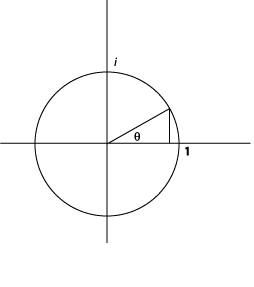Since all points on the unit circle have $|z|=1,$ by definition, multiplying any two of them together just amounts to adding the angles, so our new function $\text{Cis}\left(\theta \right)$ satisfies

But that is just how multiplication works for exponents!

That is,  ${a}^{{\theta }_{1}}{a}^{{\theta }_{2}}={a}^{{\theta }_{1}+{\theta }_{2}}$ for $a$ any constant, which strongly suggests that maybe our function $\text{Cis}\left(\theta \right)$ is nothing but some constant $a$ raised to the power $\theta ,$  that is, $\text{Cis}\left(\theta \right)={a}^{\theta }.$

It turns out to be convenient to write ${a}^{\theta }={e}^{\left(\mathrm{ln}a\right)\text{ }\theta }={e}^{A\text{ }\theta },$ say, where $A=\mathrm{ln}a.$

This line of reasoning leads us to write $\mathrm{cos}\theta +i\mathrm{sin}\theta ={e}^{A\text{ }\theta }.$

Now, for the above “addition formula” to work for multiplication, $A$ must be a constant, independent of $\theta .$  Therefore, we can find the value of $A$ by choosing $\theta$  for which things are simple.  We take $\theta$  to be very small$—$in this limit $\mathrm{cos}\theta =1,\text{\hspace{0.17em}}\text{\hspace{0.17em}}\mathrm{sin}\theta =\theta ,$, and ${e}^{A\text{ }\theta }=1+A\theta ,$ dropping terms of order ${\theta }^{2}$ and higher.

Substituting these values into $\mathrm{cos}\theta +i\mathrm{sin}\theta ={e}^{A\text{ }\theta }$ gives $A=i.$

So we find:

$\left(\mathrm{cos}\theta +i\mathrm{sin}\theta \right)={e}^{i\text{ }\theta }$

To test this result, we expand ${e}^{i\text{ }\theta }:$

$\begin{array}{c}{e}^{i\theta }=1+i\theta +\frac{{\left(i\theta \right)}^{2}}{2!}+\frac{{\left(i\theta \right)}^{3}}{3!}+\frac{{\left(i\theta \right)}^{4}}{4!}+\frac{{\left(i\theta \right)}^{5}}{5!}+...\\ =1+i\theta -\frac{{\theta }^{2}}{2!}-\frac{i{\theta }^{3}}{3!}+\frac{{\theta }^{4}}{4!}+\frac{i{\theta }^{5}}{5!}+...\\ =\left(1-\frac{{\theta }^{2}}{2!}+\frac{{\theta }^{4}}{4!}-...\right)+i\left(\theta -\frac{{\theta }^{3}}{3!}+\frac{{\theta }^{5}}{5!}-...\right)\\ =\mathrm{cos}\theta +i\mathrm{sin}\theta \end{array}$

We write $=\mathrm{cos}\theta +i\mathrm{sin}\theta$ in the last line because the series in the brackets are precisely the Taylor series for  confirming our equation for ${e}^{i\text{ }\theta }.$ Changing the sign of $\theta$ it is easy to see that

${e}^{-i\theta }=\mathrm{cos}\theta -i\mathrm{sin}\theta$

so

$\mathrm{cos}\theta =\frac{1}{2}\left({e}^{i\theta }+{e}^{-i\theta }\right)$, and  $i\mathrm{sin}\theta =\frac{1}{2}\left({e}^{i\theta }-{e}^{-i\theta }\right).$

Bottom Line: any complex number can be written:

$z=r\left(\mathrm{cos}\theta +i\mathrm{sin}\theta \right)=r{e}^{i\text{ }\theta }.$